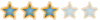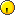Montreal Math ClubMathematics students in Montreal - Étudiants de mathématiques à MontréalHomeCalendarFAQSearchMemberlistUsergroupsRegisterLog in

 Montreal Math Club :: Mathematics :: Problem Solving ShareAuthorMessage

DescartesPosts : 100
Join date : 2009-11-05
Age : 35
Location : Right behind youSubject: 23rd Iranian Mathematical Olympiad 2008Tue Feb 23, 2010 4:12 pm Find all functions f:R^+ to R^+, such that for all x,y \in R^+ we have (x+y)f(f(x)y)=x^2f(f(x)+f(y)), where R^+ denotes the set of positive real numbers.23rd Iranian Mathematical Olympiad 2008Page 1 of 1
 Similar topics» MOA-2008-BLG-310Lb - planet in the Galactic Bulge
» Jan 23rd-24th 2016 Snow Maps Only
» Meeting, February 23rd, 7-8pm, Room 346 IMU

Permissions in this forum:You cannot reply to topics in this forumJump to: Select a forum||--Math Club|   |--Mathematical Sundays|   |--Suggestions|   |--Introduce yourself|   |--Mathematics|   |--Problem Solving|   |   |--Solved problems|   |   |   |--Calculus and Analysis|   |--Combinatorics|   |--Geometry|   |--Number Theory|   |--Probabilities and statistics|   |--Set theory and Topology|   |--Online Resources|   |--Chit chat    |--The Water Cooler    |--Mathematical poems